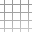#Jan Varho

## Python Raytracer in 50 Lines of Code

I've come to like the expressiveness of the Python language, though I dislike some of the other features. Here's a 50 line raytracer that renders scenes of colored spheres to a PNG file:

import math from PIL import Image

def sub(v1, v2): return [x-y for x,y in zip(v1, v2)] def dot(v1, v2): return sum([x*y for x,y in zip(v1, v2)]) def norm(v): return [x/math.sqrt(dot(v,v)) for x in v]

def trace_sphere(r, s, tmin, color): sr = sub(s, r) dsr = dot(sr, r)

d = dsr*dsr - dot(sr,sr) + s if d < 0: return d = math.sqrt(d)

t1 = dsr + d if t1 < 0: return

t2 = dsr - d if t2 > tmin: return

if t2 < 0: tmin = t1 else: tmin = t2 color[:] = [s]

def trace_ray(ray, spheres): color = [(0, 0, 0)] tmin =  for s in spheres: trace_sphere(ray, s, tmin, color) return color

red = (255,0,0) blue = (0,0,255) spheres = ( ((0,0,100), 100.0, red), ((0,-5,50), 20.0, blue) )

w, h = 200, 200

image = Image.new("RGB", (w, h)) pix = image.load()

for x in range(w): for y in range(h): ray = ( (0,0,0), norm(((x-w/2.0)/w, (y-h/2.0)/w, 1)) ) pix[x,y] = trace_ray(ray, spheres)

image.save("out.png")

Ok, it's more like 30 significant likes to tell the truth.